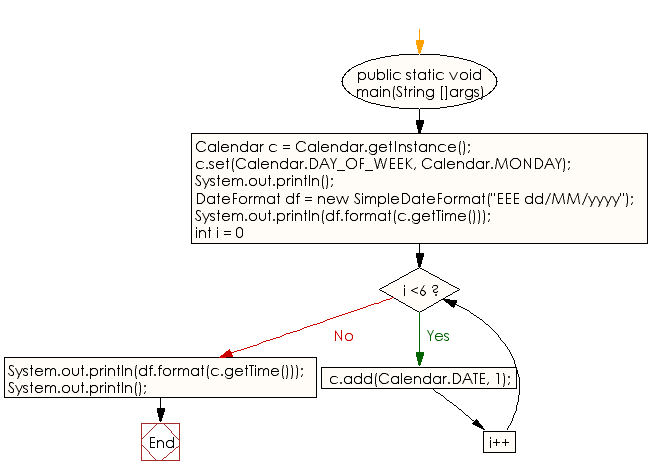﻿ Java exercises: Calculate the first and last day of each week - w3resource# Java DateTime, Calendar Exercises: Calculate the first and last day of each week

## Java DateTime, Calendar: Exercise-9 with Solution

Write a Java program to calculate the first and last day of each week.

Sample Solution:

Java Code:

``````import java.util.*;
import java.time.*;
import java.text.*;

public class Exercise9 {

public static void main(String []args){
// Get calendar set to current date and time
Calendar c = Calendar.getInstance();

// Set the calendar to monday of the current week
c.set(Calendar.DAY_OF_WEEK, Calendar.MONDAY);

System.out.println();
// Print dates of the current week starting on Monday
DateFormat df = new SimpleDateFormat("EEE dd/MM/yyyy");
System.out.println(df.format(c.getTime()));
for (int i = 0; i <6; i++) {
}
System.out.println(df.format(c.getTime()));
System.out.println();
}
}
```
```

Sample Output:

```Mon 19/06/2017
Sun 25/06/2017
```

N.B.: The result may varry for your system date and time.

Pictorial Presentation:Flowchart:Java Code Editor:

Improve this sample solution and post your code through Disqus

What is the difficulty level of this exercise?

﻿

## Java: Tips of the Day

Easiest way to convert a List to a Set in Java:

```Set<Foo> foo = new HashSet<Foo>(myList);
```

Ref: https://bit.ly/3qIJSTZ